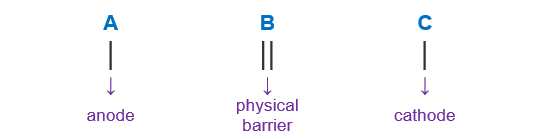# Problem: The voltage generated by the zinc concentration cell described by, Zn(s)|Zn2+(aq, 0.100 M)||Zn2+(aq, ? M)|Zn(s) is 23.0 mV at 25°C. Calculate the concentration of the Zn2 + (aq) ion at the cathode.

###### FREE Expert Solution

The Nernst Equation at 25°C:

Ecell = cell potential under non-standard conditions
cell = standard cell potential
n = number of e- transferred
Q= reaction quotient = [products]/[reactants]

When writing a cell notation, we use the following format – “as easy as ABCGet the overall reaction by balancing the number of electrons transferred then adding the oxidation half-reaction and reduction half-reaction.

Zn2+(aq) + 2 e- → Zn(s)

Zn(s) → Zn2+(aq) + 2 e-
__________________

Zn(s) + Zn2+(aq)→ Zn2+(aq) + Zn(s)

# of e- transferred = n = 2 e-

100% (62 ratings)###### Problem Details

The voltage generated by the zinc concentration cell described by,

Zn(s)|Zn2+(aq, 0.100 M)||Zn2+(aq, ? M)|Zn(s)

is 23.0 mV at 25°C. Calculate the concentration of the Zn2 + (aq) ion at the cathode.